### IMO Shortlist 1994 problem G2

Kvaliteta:
Avg: 0,0
Težina:
Avg: 6,0$ABCD$ is a quadrilateral with$BC$ parallel to$AD$.$M$ is the midpoint of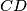$CD$,$P$ is the midpoint of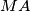$MA$ and$Q$ is the midpoint of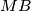$MB$. The lines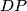$DP$ and$CQ$ meet at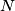$N$. Prove that$N$ is inside the quadrilateral$ABCD$.
Izvor: Međunarodna matematička olimpijada, shortlist 1994